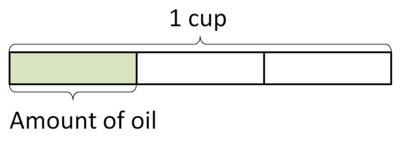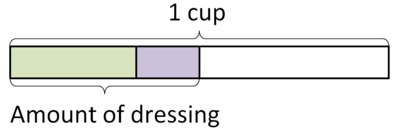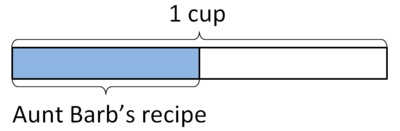##### Engage your students with effective distance learning resources. ACCESS RESOURCES>>

Alignments to Content Standards: 5.NF.A.2 5.NF.B.7.c

• $\frac13$ cup olive oil
• $\frac16$ cup balsamic vinegar
• a pinch of herbs
• a pinch of salt
Makes 6 servings
1. How many cups of salad dressing will this recipe make? Write an equation to represent your thinking. Assume that the herbs and salt do not change the amount of dressing.
2. If this recipe makes 6 servings, how much dressing would there be in one serving? Write a number sentence to represent your thinking.

## IM Commentary

The purpose of this task is to have students add fractions with unlike denominators and divide a unit fraction by a whole number. This accessible real-life context provides students with an opportunity to apply their understanding of addition as joining two separate quantities. Additionally, the context presents a "how many groups" division problem where a unit fraction should be divided into 6 equal groups.

The Standards for Mathematical Practice focus on the nature of the learning experiences by attending to the thinking processes and habits of mind that students need to develop in order to attain a deep and flexible understanding of mathematics. Certain tasks lend themselves to the demonstration of specific practices by students. The practices that are observable during exploration of a task depend on how instruction unfolds in the classroom. While it is possible that tasks may be connected to several practices, only one practice connection will be discussed in depth. Possible secondary practice connections may be discussed but not in the same degree of detail.

This particular task helps illustrate Mathematical Practice Standard 4, Model with mathematics. Students apply the mathematics they know to solve problems arising in everyday life. For this problem, fifth graders apply their understanding of addition as joining two separate quantities along with their understanding of division of a unit fraction by a whole number. The addition of fractions with unlike denominators can be shown symbolically or by creating a bar diagram depicting 1/3 cup of olive oil and 1/6 cup of balsamic vinegar. In order to make sense of these quantities, students will subdivide the 1/3 cup of olive oil into sixths. Then they can visually see that the two ingredients total 3/6 of a cup which is ½ of one whole cup.  This same method may be used to divide the ½ cup of salad dressing into 6 equal portions. In each instance, students use the mathematics they know to find a solution pathway and then capture their thinking in an equation.  By using the mathematical model of an equation to represent the real life situation described in the task students exercise their ability to model with the mathematics that they know.

## Attached Resources

• Lesson Plan: Cooking Time 4
• ## Solution

1. The total amount of dressing can be found by adding the oil and vinegar (since the other two ingredients won't change the amount). Here is a picture that represents the amount of oil:and here is a picture that represents the amount of vinegar:We can show the sum like this:It is hard to tell by looking at it what fraction of the whole cup this is, but if we divide each of the thirds into two pieces, we can see how much a third is in terms of sixths:Now we can show the sum in a way that makes it much easier to see what fraction of the whole cup it is:If we look carefully at the picture, we can see that $\frac36 = \frac12$.

We can write this whole process up symbolically like this:

\begin{align} \frac13 + \frac16 & = \frac26 + \frac16 \\ & = \frac36 \\ & = \frac12 \end{align}
So the total amount of dressing will be $\frac12$ cup.

2. There is $\frac12$ cup of dressingand 6 servings, so each serving is: $$\frac12 \div 6$$ cups of dressing. We can picture this by dividing the $\frac12$ cup of dressing into 6 equal parts:To see what fraction of a cup this is, we should divide the other $\frac12$ cup into 6 equal parts:so the whole cup is divided into 12 equal parts. From the picture, we can see: $$\frac12 \div 6 = \frac{1}{12}$$ So each serving will be $\frac{1}{12}$ cup of salad dressing.